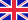•# Combinatorics and the Feynman Identity

Autor: MINGSHR LIN

The book investigated the applications of combinatorics and graph theory in the analysis of the Feynman Identity of the partition function of the two dimensional Ising Model. Chapter one gives a general introduction to the partition function of the Ising... Viac o knihe

1 kus - skladom u vydavateľa Posielame do 7-10 dní

42.84 €

bežná cena: 50.40 €

## O knihe

The book investigated the applications of combinatorics and graph theory in the analysis of the Feynman Identity of the partition function of the two dimensional Ising Model. Chapter one gives a general introduction to the partition function of the Ising Model and the Feynman Identity in the language of graph theory.Chapter two describes and proves combinatorially the Feynman Identity in a special case when there is only one vertex and multiple loops. Chapter three introduces a new way to calculate the number of cycles in a directed graph, along with its application in the special case discussed in chapter two to derive the analytical expression of the number of non-periodic cycles. Chapter four comes back to the general form of the Feynman Identity and several combinatorial identities are derived by introducing special conditions of the graph and applying the Feynman Identity under the condition. Chapter five concludes the work by summarizing the main idea in each chapter and provides insight for generalizations in three dimensional Ising Model.

• Vydavateľstvo: VDM Verlag
• Rok vydania: 2009
• Formát: Paperback
• Rozmer: 221 x 149 mm
• Jazyk: Anglický jazyk
• ISBN: 9783639178210

Generuje redakčný systém BUXUS CMS spoločnosti ui42.